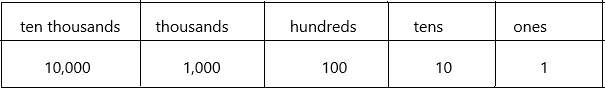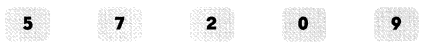# Math in Focus Grade 4 Chapter 1 Practice 1 Answer Key Numbers to 10,000

Go through the Math in Focus Grade 4 Workbook Answer Key Chapter 1 Practice 1 Numbers to 10,000 to finish your assignments.

## Math in Focus Grade 4 Chapter 1 Practice 1 Answer Key Numbers to 10,000

Write each number in standard form.

Example.
Seventy-two thousand, four hundred sixty 72,460

Question 1.
seventy thousand, eight hundred twenty-three ____
7 is in the ten thousand place
0 is in the thousands place
8 is in the hundreds place
2 is in the tens place
3 is in the one’s place.
By using a place chart we can easily write the number:According to the place value chart write the number: 70,823

Question 2.
sixty-two thousand, four hundred eighteen ____
6 is in the ten thousand place
2 is in the thousands place
4 is in the hundreds place
1 is in the tens place
8 is in the one’s place.

Question 3.
ninety-seven thousand, four hundred ____
9 is in the ten thousand place
7 is in the thousands place
4 is in the hundreds place
0 is in the tens place
0 is in the one’s place.

Question 4.
thirty thousand, eleven ____
3 is in the ten thousand place
0 is in the thousands place
0 is in the hundreds place
1 is in the tens place
1 is in the one’s place.

Write each number in word form.

Example
56,548 fifty-six thousand, five hundred forty-eight

Question 5.
12,021 ______
Explanation:
– On beginning with the first digit that is 1. It is present in the place of a ten thousand – 10,000
– The next number to the right side is thousands place – two thousand
– The comma present on the rightmost side represents – twenty-one
– 12,021 in word format is twelve thousand, twenty-one.

Question 6.
70,009 ______
Explanation:
Explanation:
– On beginning with the first digit that is 1. It is present in the place of a ten thousand – 70,000
– The comma present on the rightmost side represents – nine
– 70,009 in word format is seventy thousand nine

Question 7.
40,807 ______
Explanation:
– On beginning with the first digit that is 1. It is present in the place of a ten thousand – 40,000
– The comma present on the rightmost side represents – eight hundred seven
– 40,807 in word format is forty thousand eight hundred seven.

Count on and fill in the blanks.

Question 8.
81,000 82,000 83,000 ____ ____
A list of numbers that follow a certain sequence is known as patterns or number patterns. The different types of number patterns are algebraic or arithmetic patterns, geometric patterns, Fibonacci patterns and so on. Now, let us take a look at the three different patterns here.
Arithmetic pattern: The arithmetic pattern is also known as the algebraic pattern. In an arithmetic pattern, the sequences are based on the addition or subtraction of the terms. If two or more terms in the sequence are given, we can use addition or subtraction to find the arithmetic pattern.
The above-given sequence, 81,000 82,000 83,000 ____ ____ . Now, we need to find the missing term in the sequence.
Here, we can use the addition process to figure out the missing terms in the patterns.
In the pattern, the rule used is “Add 1000 to the previous term to get the next term”.
In the given above, take the second term (82,000). If we add “1000” to the second term (82,000), we get the third term 83,000.
Similarly, we can find the unknown terms in the sequence.
First missing term: The previous term is 83,000. Therefore, 83,000+1000= 84,000.
Second missing term: The previous term is 84,000. So, 84,000+1000 = 85,000.
Hence, the complete arithmetic pattern is 81,000 82,000 83,000  84,000  85,000.

Question 9.
30,000 40,000 50,000 ___ ___
A list of numbers that follow a certain sequence is known as patterns or number patterns. The different types of number patterns are algebraic or arithmetic patterns, geometric patterns, Fibonacci patterns and so on. Now, let us take a look at the three different patterns here.
Arithmetic pattern: The arithmetic pattern is also known as the algebraic pattern. In an arithmetic pattern, the sequences are based on the addition or subtraction of the terms. If two or more terms in the sequence are given, we can use addition or subtraction to find the arithmetic pattern.
The above-given sequence, 30,000 40,000 50,000 ___ ___ . Now, we need to find the missing term in the sequence.
Here, we can use the addition process to figure out the missing terms in the patterns.
In the pattern, the rule used is “Add 10,000 to the previous term to get the next term”.
In the given above, take the second term (40,000). If we add “1000” to the second term (40,000), we get the third term of 50,000.
Similarly, we can find the unknown terms in the sequence.
First missing term: The previous term is 50,000. Therefore, 50,000+10,000= 60,000.
Second missing term: The previous term is 60,000. So, 60,000+1000 = 70,000.
Hence, the complete arithmetic pattern is 30,000 40,000 50,000   60,000   70,000

Question 10.
10,000 15,000 20,000 ___ ____
A list of numbers that follow a certain sequence is known as patterns or number patterns. The different types of number patterns are algebraic or arithmetic patterns, geometric patterns, Fibonacci patterns and so on. Now, let us take a look at the three different patterns here.
Arithmetic pattern: The arithmetic pattern is also known as the algebraic pattern. In an arithmetic pattern, the sequences are based on the addition or subtraction of the terms. If two or more terms in the sequence are given, we can use addition or subtraction to find the arithmetic pattern.
The above-given sequence, 10,000 15,000 20,000 ___ ____ . Now, we need to find the missing term in the sequence.
Here, we can use the addition process to figure out the missing terms in the patterns.
In the pattern, the rule used is “Add 5,000 to the previous term to get the next term”.
In the given above, take the second term (15,000). If we add “5000” to the second term (15,000), we get the third term of 20,000.
Similarly, we can find the unknown terms in the sequence.
First missing term: The previous term is 20,000. Therefore, 20,000+5,000= 25,000.
Second missing term: The previous term is 25,000. So, 25,000+5000 = 30,000.
Hence, the complete arithmetic pattern is 10,000 15,000 20,000  25,000   30,000

Example
two thousand, five hundred twelve 2,512

Question 11.
sixty-one thousand, ____ ___ 1,001
The word form is sixty-one thousand, one
Explanation:
– On beginning with the first digit that is 6. It is present in the place of a ten thousand – 61,000
– The comma present on the rightmost side represents – one
– 61,001 in word format is sixty-one thousand, one.
The standard form is 61,001.

Question 12.
twenty-four ____, three hundred ten 24,3__0
The word form is twenty-four thousand, three hundred ten.
Explanation:
– On beginning with the first digit that is 2. It is present in the place of a ten thousand to thousands – 24,000
– The comma present on the rightmost side represents – three hundred one
– 24,310 in word format is twenty-four thousand, three hundred ten.
The standard form is 24,310.

Question 13.
forty-five thousand, ____ hundred six 4 __,206
Explanation:
– On beginning with the first digit that is 4. It is present in the place of a ten thousand to thousands – 45,000
– The comma present on the rightmost side represents – two hundred six
– 45,206 in word format is forty-five thousand, two hundred six.
The standard form is 45,206.

Question 14.
thirty-six thousand, one hundred. _____ 36,89
Explanation:
– On beginning with the first digit that is 3. It is present in the place of a ten thousand to thousands – 36,000
– The comma present on the rightmost side represents – one hundred
– 36,100 in word, format is thirty-six thousand, one hundred
The standard form is 36,100

Make each 5-digit number using all the cards. Do not begin a number with ‘0’.Question 15.
An odd number: _______________________________________
Generally, the even and odd numbers are defined as follows:
Definition 1: “Even numbers are those numbers which are divisible by 2 and odd numbers which are not divisible by two”.
Definition 2: “Even numbers are those which when divided by 2 leaves no remainder or as 0 and Odd numbers are those numbers which when divided by 2 leaves a remainder of 1”.
How to check a number is even or odd:
– As we know now that “Even numbers those numbers which end with 0,2,4,6,8 and odd numbers are those numbers which end with 1,3,5,7,9.”
– So, first, look at the number in the one’s place. This single number will tell whether the entire number is odd or even.
Here the above number is 52,907
When it divided by 2 it leaves remainder 1. So it is odd number.

Question 16.
An even number: ________________________________________
Generally, the even and odd numbers are defined as follows:
Definition 1: “Even numbers are those numbers which are divisible by 2 and odd numbers which are not divisible by two”.
Definition 2: “Even numbers are those which when divided by 2 leaves no remainder or as 0 and Odd numbers are those numbers which when divided by 2 leaves a remainder of 1”.
How to check a number is even or odd:
– As we know now that “Even numbers those numbers which end with 0,2,4,6,8 and odd numbers are those numbers which end with 1,3,5,7,9.”
– So, first, look at the number in the one’s place. This single number will tell whether the entire number is odd or even.
Here the above number is 25,970
When it is divided by 2 it leaves the remainder 0. So it is an even number.

Question 17.
A number with zero in the hundreds place: _______________________________
By using the above numbers we can write the number having 0 in the hundreds place.
5 hold the ten thousand place.
2 hold the thousands place.
0 holds the hundreds place
9 hold the tens place.
7 holds the one’s place.

Question 18.
A number beginning with the greatest digit: _______________________________
By using the above-given numbers are have the highest digit.
that digit is 9.
So we can write with 9 keeping in ten thousand place.
9 hold the ten thousand place.
5 hold the thousands place.
7 holds the hundreds place
2 hold the tens place.
0 holds the one’s place.

Question 19.
A number with 2 in the tens place and 5 in the ones place: _______________________________
9 hold the ten thousand place.
0 hold the thousands place.
7 holds the hundreds place
2 hold the tens place.
5 holds the one’s place.

Question 20.
A number ending with 7: _______________________________